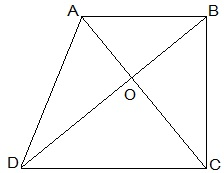Guru

# Diagonals AC and BD of a quadrilateral ABCD intersect at O in such a way that ar(△AOD) = ar(△BOC). Prove that ABCD is a trapezium. Q.15

• 0

Hello sir i want to know the best solution of the question from exercise 9.3of math of Areas of Parallelograms and Triangles chapter of class 9th give me the best and easy for solving this question how i solve it of question no.15 Diagonals AC and BD of a quadrilateral ABCD intersect at O in such a way that ar(△AOD) = ar(△BOC). Prove that ABCD is a trapezium.

Share

1.Given,

ar(△AOD) = ar(△BOC)

To Prove,

ABCD is a trapezium.

Proof:

ar(△AOD) = ar(△BOC)

⇒ ar(△AOD) + ar(△AOB) = ar(△BOC)+ar(△AOB)